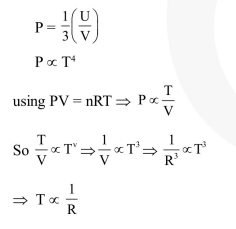# Consider a spherical shell of radius

Question:

Consider a spherical shell of radius $\mathrm{R}$ at temperature $\mathrm{T}$. The black body radiation inside it can be considered as an ideal gas of photons with internal energy per unit volume $\mathrm{u}=\frac{\mathrm{U}}{\mathrm{V}} \propto \mathrm{T}^{4}$ and pressure $\mathrm{p}=\frac{1}{3}\left(\frac{\mathrm{U}}{\mathrm{V}}\right)$. If the shell now undergoes an adiabatic expansion the relation between $\mathrm{T}$ and $\mathrm{R}$ is -

1. $T \propto \frac{1}{R}$

2. $\mathrm{T} \propto \frac{1}{\mathrm{R}^{3}}$

3. $\mathrm{T} \propto \mathrm{e}^{-\mathrm{R}}$

4. $\mathrm{T} \propto \mathrm{e}^{-3 \mathrm{R}}$

Correct Option: 1

Solution: